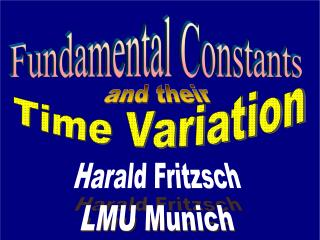# Fundamental Constants - PowerPoint PPT PresentationDownload PresentationFundamental Constants

Fundamental ConstantsDownload Presentation## Fundamental Constants

- - - - - - - - - - - - - - - - - - - - - - - - - - - E N D - - - - - - - - - - - - - - - - - - - - - - - - - - -
##### Presentation Transcript

1. Time Variation Fundamental Constants and their Harald Fritzsch LMU Munich

2. - fundamental constants the problem of modern science

3. particle physics nuclear … atomic … laser … solid state … astro … cosmology fundamental constants =>

4. ==> chemistry, biology, ...

5. - Fundamental constants Standard Model

6. - Standard Model gauge theories

7. - first gauge theory QED

8. - QED 2 fundamental constants

9. 1964 ===> electroweak gauge theory U(1) x SU(2)

10. gauge theory of the Strong Interactions

11. - M. Gell-Mann G. Zweig M. Gell-Mann G. Zweig 1964 quarks

12. - ( ) u ( ) p d n s electric charge:

13. - 1971 color q=> q q q SU(3,c)

14. - - Hadrons - white states

15. - 1971 - 1972 QCD Fritzsch & Gell-Mann

16. 8 gluons

17. - 1973: Standard Model SU(3) x SU(2) x U(1) 2 ==> 28

18. problem ===> 28 fundamental constants

19. Newtons constant G 1 fine structure constant 1coupling constant of strong interaction 1coupling constant of weak interaction 1mass of W boson 1mass of Higgs boson 1masses of 6 quarks and 6 leptons 12 flavor mixing of quarks 4flavor mixing of leptons 6 - 28

20. 28

21. (22 related to fermion masses)

22. - MeV masses 100 10 1

23. - Arnold Sommerfeld, 1916 fine-structure constant

24. - electrodynamics + relativity + quantum theory

25. QED

26. partial screening + - + + - - - -

27. fine-structure constant ==> function of energy +

28. - LEP: ~ 1/127

29. - nucleon mass fundamental constant ? ==> QCD

30. - quark masses ==> 0 mass <=> field energy

31. dimensional transmutation

32. nucleon mass ( quark masses => 0 =

33. Experiments: ~ 250 MeV mass <==> confined field energy

34. real world: QCD u d s QED M (proton) = 860 + 21 + 19 + 36 + 2

35. Standard Model: fundamental constants in our universe universal

36. Are the fundamental constants functions of time and space?

37. - function of energy

38. - ? function of time?

39. About 1.8 billion years ago, in Gabon, Westafrika. Natural Reactor, which operated about 100 million years. High concentration of uranium 3.7% U 235 at that time (today 0.72 %) Moderator: water from river Oklo Oklo Phenomen

40. discovery: 1972 Natural reactor ( output: ~ 100 kw )

41. Oklo zones

42. - samarium: neutron capture Sm(149) + n => Sm(150) + photon cross section about 80 kb nuclear resonance: E = 0.0973 eV

43. - change of resonance position less than 0.1 eV in 2 billion years constraint for fine-structure constant: ( Dyson, Damour)

44. Change of alpha per year must be less than per year (if no other parameters change) ==>constraint questionable -16 10

45. - limits on time variation of constantes, related to stable matter: fine-structure constant mass of electron QCD scale quark masses

46. time variation of QCD scale:

47. u / d - quarks ~ 20 MeV of proton mass